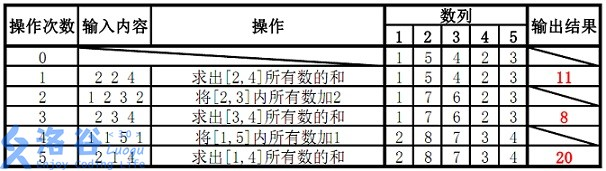# 线段树（懒标记）总结

/ 1评 / 3

——维基百科

## 例题

1.将某区间每一个数加上x
2.求出某区间每一个数的和

### 输入输出样例

5 5
1 5 4 2 3
2 2 4
1 2 3 2
2 3 4
1 1 5 1
2 1 4


11
8
20


### 说明

（数据已经过加强^_^，保证在int64/long long数据范围内）## 线段树的定义和构造

inline void build(int p,int tl,int tr){
tag[p]=0;
if (tl==tr) {tree[p]=a[tl];return;}
int mid=((tr-tl)>>1)+tl;
build((p<<1)  ,tl,mid  );
build((p<<1)+1,mid+1,tr);
tree[p]=tree[p<<1]+tree[(p<<1)+1];
}


## 完整代码（注释版）

#include<cstdio>
#include<cstring>
#include<iostream>
using namespace std;
const int maxn=2000005;
int n,m;
long long a[maxn],tree[maxn],tag[maxn];
int ret=0,f=1;char ch=getchar();
while (ch<'0'||ch>'9') {if (ch=='-') f=-1;ch=getchar();}
while (ch>='0'&&ch<='9') ret=ret*10+ch-'0',ch=getchar();
return ret*f;
}
long long ret=0,f=1;char ch=getchar();
while (ch<'0'||ch>'9') {if (ch=='-') f=(long long)-1;ch=getchar();}
while (ch>='0'&&ch<='9') ret=ret*(long long)10+(long long)(ch-'0'),ch=getchar();
return (long long)ret*f;
}
inline void build(int p,int tl,int tr){  //构造Segment Tree
tag[p]=0;
if (tl==tr) {tree[p]=a[tl];return;}
int mid=((tr-tl)>>1)+tl;
build((p<<1)  ,tl,mid  );
build((p<<1)+1,mid+1,tr);
tree[p]=tree[p<<1]+tree[(p<<1)+1];
}
inline void push_down(int tl,int tr,int p){  //push_down函数负责向下传递，将存储的tag数组用于修正
int mid=((tr-tl)>>1)+tl;
tree[p<<1]+=(long long)tag[p]*(mid-tl+1);  //只需要修正一对左右儿子，不需要一直传递到叶节点
tag[p<<1]+=tag[p];  //因为没有往下修正，所以要把儿子的tag累计上
tree[(p<<1)+1]+=(long long)tag[p]*(tr-mid);
tag[(p<<1)+1]+=tag[p];
tag[p]=0;
}
inline void update(int sl,int sr,int tl,int tr,long long delta,int p){
if (sl<=tl&&sr>=tr){  //p管辖的区间在修改范围内
tree[p]+=delta*(long long)(tr-tl+1);
tag[p]+=delta;  //tag数组记录剩下的未向下传递的，实现“lazy tag”
return;
}
push_down(tl,tr,p);  //如果没有完全包含p管辖的区间，则说明应该向下“分割”，所以应该用tag数组先修正下面的tree[]
int mid=((tr-tl)>>1)+tl;  //分割[tl,tr]区间
if (sl<=mid) update(sl,sr,tl,mid,delta,p<<1);
if (mid<sr)  update(sl,sr,mid+1,tr,delta,(p<<1)+1);
tree[p]=tree[p<<1]+tree[(p<<1)+1];  //向上累计
}
inline long long query(int sl,int sr,int tl,int tr,int p){  //查询操作
if (sl<=tl&&sr>=tr) return tree[p];  //如果完全包含p所管辖的区间则直接返回tree[p]
long long ret=0;  //否则就“分割”
push_down(tl,tr,p);  //先要把当前的tag用于修正下面两个儿子，这样才能保证下面query用到的tree[]是正确的
int mid=((tr-tl)>>1)+tl;
if (sl<=mid) ret+=(long long)query(sl,sr,tl,mid,p<<1);
if (mid<sr)  ret+=(long long)query(sl,sr,mid+1,tr,(p<<1)+1);
return ret;
}
int main(){
freopen("tree.in","r",stdin);
freopen("tree.out","w",stdout);
build(1,1,n);
for (int i=1;i<=m;i++){
if (c==1){
update(x,y,1,n,z,1);
} else
if (c==2){
printf("%lld\n",query(x,y,1,n,1));
}
}
return 0;
}


## 参考

1.StungYep说道：